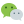# C 语言自增自减运算辨析

c语言中，自增(++)和自减(–)运算是很有c语言“感觉”的一种运算符，但是在实际编写中，尤其对初学者或者很久没接触它的，会对它的原理和运算结果产生混淆，接下来做详细辨析。

# 自增运算（++）

``````((3+a)*sqrt(3)/(a%2))=((3+a)*sqrt(3)/(a%2))+1;

/*简化后*/
((3+a)*sqrt(3)/(a%2))++;
``````

## 辨析

``````#include<stdio.h>
int main()
{
int a = 1, b = 1, a2, b2;
a2 = a++;
b2 = ++b;
printf("a = %d, b = %d, a2 = %d, b2 = %d", a, b, a2, b2);
}
``````

``````a = 2, b = 2, a2 = 1, b2 = 2
``````

`b = a++` 是先赋值后运算，即先 `b=a`，然后 `a++` `b = ++a` 是先运算后赋值，即先 `++a`，然后 `++a`

# 自减运算

### 评论：微信公众号：程序骑士